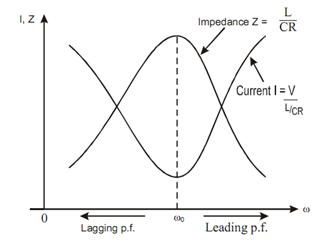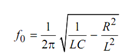## Resonance Curve Assignment Help

Assignment Help: >> Parallel Resonance - Resonance Curve

Resonance Curve:

As admittance is minimum or impedance is maximum at resonance frequency ωC, so current I is also minimum at parallel resonance.Figure: Resonance Curve for Parallel Resonance

Properties of Resonance of parallel RLC circuit

1. Power factor is unity.

2. Current at resonance is [V / (L / CR)] and is in phase with the applied voltage. The value of current at resonance is minimum.

3. Net impedance at resonance of the parallel circuit is maximum and equal to (L / CR) Ω.

4. The admittance is minimum and the net susceptance is zero at resonance.

5. The resonance frequency of this circuit is specified by